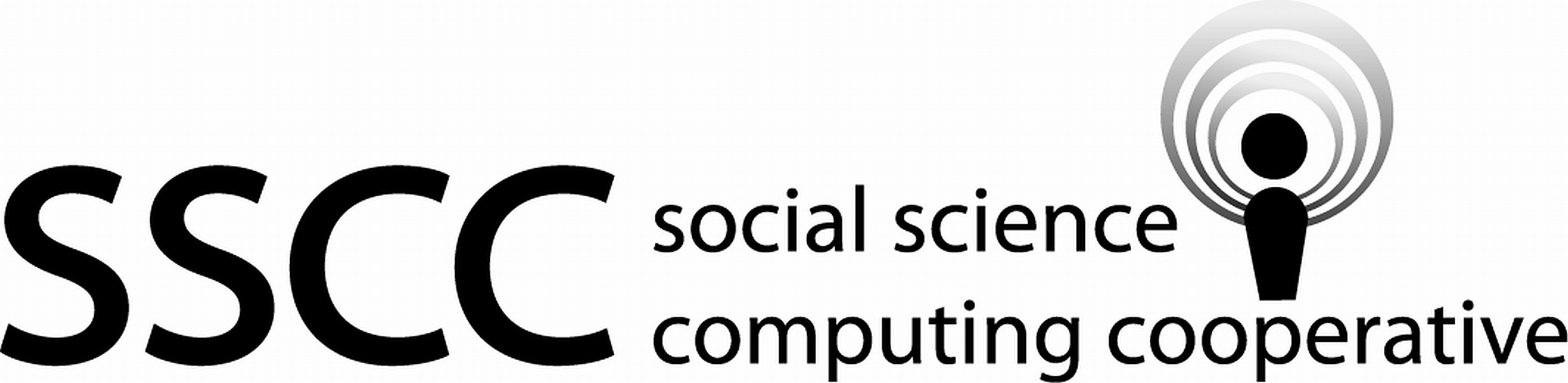Supporting Statistical Analysis for Research

## 2.1 Data frames

1. Create a data frame with three observations of two variables. Name the variables `x1` and `x2`. Make up numbers for the values of the observed variables.

``library(tidyverse)``
``````my_data <-
tibble(
x1 = c(1, 2, 3),
x2 = c("c", "b", "a")
)``````
2. Using any of the functions/methods from the discourse, display the number of observations and variables of the data frame.

``glimpse(my_data)``
``````Observations: 3
Variables: 2
\$ x1 <dbl> 1, 2, 3
\$ x2 <chr> "c", "b", "a"``````

Or

``head(my_data)``
``````# A tibble: 3 x 2
x1 x2
<dbl> <chr>
1     1 c
2     2 b
3     3 a    ``````